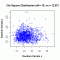I have forgotten

•http://facebook.com/
•https://www.google.com/accounts/o8/id
•https://me.yahoo.com

# Chisquared

#### CDF

Evaluates the chi-squared CDF.
 double CDF (double r, double x, bool upper = false)

#### CDF Inv

Inverse of the complemented chi-square distribution
 double CDF_inv (double r, double y)

#### Random Sample

Generates random numbers following a Chi-Square distribution.
 RandomSample::RandomSample unknown (int df, double noncen, double s) RandomSample::~RandomSample unknown () void RandomSample::init (int df, double noncen) double RandomSample::genReal () void RandomSample::Build1 (double d) void RandomSample::Build2 (double alphax) void RandomSample::BuildExp () double RandomSample::Density (double x) double RandomSample::Gen1 () double RandomSample::Gen2 () double RandomSample::GenExp () double sample (int df, double noncen, double seed=0)